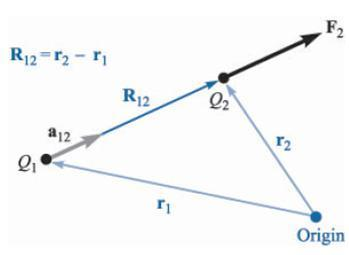# The Coulombs Law

Columbs law state that the force between two small objects seperated in a vaccum by distance much greater that the size of each object is proportional to the product of charges on them and inversely proportional to square of distance between them.

where Q1 and Q2 are positive or negative charge quantity measured in columbs and R is the distance between them measured in meters. Here k is proportionality constant given as:

Again another constant € is called permittivity of free space. Measured in Farad per meter(F/m).

The above quantity is not dimensionless. For coulombs law dimensions are C2/N.m2 . This means Farad has units C2/N.m .This makes are coulombs law as:

VECTOR FORM OF COULOMBS LAW:Vector form of Coulombs law

Let us say charges Q1 and Q2 are like charges distance of each from origin be r1 and r2 respectively. As F2 is in direction of R12. The vector R12 is cleary
R12 = r2 – r1.
Therefore the vector form of coulombs law is:

Where a12 is a unit vector in direction of R12 or we can say:

Let us finish this topic with an example.
Example: let umm. There be 2 charges say Q1 = 3 x 10^(-4) C located at say M(1,2,3) and Q2 = 10^(-4) C located at say L(2,0,5). We require to find force exerted by Q2 on Q1.
Answer: Let F2 = force exerted by Q2 on Q1.
Vector R12 = r2 – r1 = (2 – 1)i + (0 – 2)j + (5 – 3)k = i – 2j + 2k
This gives us |R12| = 3
Which again gives as unit vector

Therefore our F2

F2 = 10i – 20j + 20k N ans.
Therefore we found out F2 that is force of Q2 on Q1. Similary we can find F1 that is force of Q1 on Q2. Interestingly as both charges are like charges. We will find out that

Coulombs law is linear. We can see that multiplying Q1 by some factor n. We find that the force between the 2 charges also get multiplied by factor n.
Another thing is that the force on the charge in presence of the other charges is sum of forces on that charge due to each of the other charges acting alone.